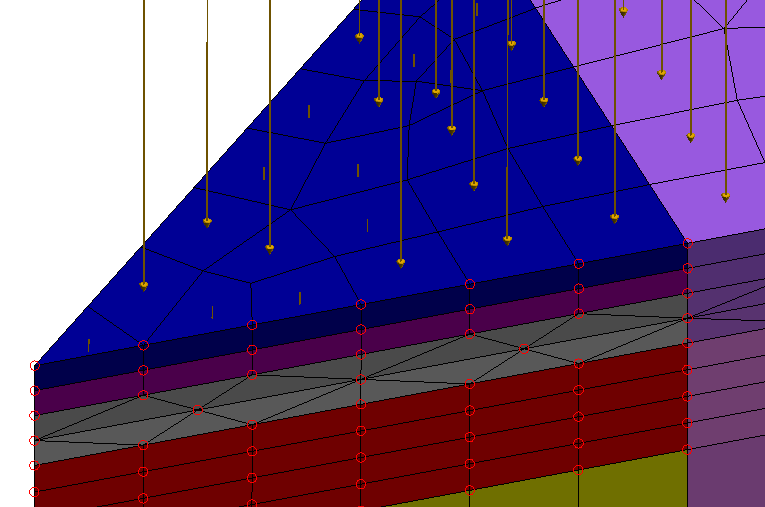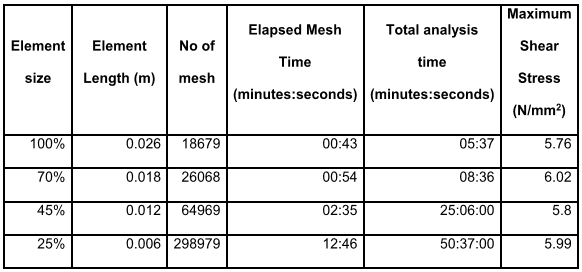Figure 1: Mesh, loads and constraints, ready for analysis
© Glew Engineering Consulting, inc. 2016

Welcome again to our series on finite element analysis (FEA).  In the last blogs, I covered steps on setting up a computer-aided design (CAD) model and how to set up the mesh and boundary conditions, the most crucial steps in FEA simulation.  In this blog, I’ll look at the actual simulation and analysis, which can be the most time-consuming stage in the process.As a reminder, for an example I’ve been using a recent project we worked on involving punching shear in reinforced concrete.  We were examining the effectiveness of reinforcing a column-supported concrete slab against the possibility of that column punching through the concrete.

# Types of Analysis

Once the CAD model has been created, the materials properties defined, the mesh generated, and the loads and boundary conditions set (leaving a model looking like Figure 1), the model is set for analysis.  There are a few different types of analysis software that FEA programs can utilize, based on the specific scenario being modeled.

• Linear: Linear analysis is useful for simpler systems with small deflections, linear elastic properties, and unchanging loads and boundary conditions. This type of analysis runs faster than nonlinear types, since it simplifies many of the parameters in the governing equations used to calculate results. However, those same simplifications can lead to invalid results if the model deforms too much under load.  Experienced engineers may recall the difference between “engineering strain” (e = ΔL/L) and “true strain” (ε = ln(1 + e)); the two are equivalent for small values of ΔL, but quickly diverge as the deformation increases.
• Nonlinear: Nonlinear analysis types encompass more complicated problems involving motion, nonlinear elastic or plastic materials, time-dependent changes to loads and constraints, or large deflections and changes in load direction due to those displacements. Nonlinear analysis can be more complicated to set up and take longer to run, but it is necessary for certain scenarios. The physics of certain problems are inherently nonlinear, such as radiation and fluid dynamics.
• Thermal: Thermal analysis calculates temperature and heat fluxes due to thermal loads, either for steady-state systems or time-variant systems. The latter can be used for materials that change phases between solid and liquid.
• Electrostatic: Electrostatic analysis can calculate current, electric field, and voltage distribution based on induced electrical parameters.

# Time-Consuming Analysis

At the most basic level, all of the above types of analysis essentially work the same way.  Each of the thousands or hundreds of thousands of elements in a model is represented by a series of partial differential equations representing their stress and strain.  While partial differential equations are difficult to solve manually, a computer can solve them rapidly by using the value at one boundary, checking the error, and iterating through again until the error is minimized.  It solves the equations for one element, then moves on the next element using the solutions from the last one.  The computer iterates through the entire model in this fashion, so the time begins to add up quickly when analyzing a complex model.  The most complex models can require hours or days to iterate through every element until the accumulated error has been sufficiently reduced.

# Reinforced Concrete Problem

For example, in our reinforced concrete problem we initially attempted a linear analysis, to ensure that the system was responding to the loads and constrains correctly.  However, we quickly realized that linear models would not suffice, considering the complex behavior of concrete under loads (it can withstand a lot of compressive force but fails very quickly under tension), the added complexity of adding two layers of rebar reinforcement, and finally the possibility of large deflections over such a big model.  We achieved our final results using Autodesk Simulation’s “Mechanical Event Simulator (MES) with nonlinear materials” simulation mode.  MES was designed to simulate dynamic events that occur over a period of time or with significant movement or deformation, like our meters-long section of concrete flexing under its own weight.

# Mesh Refinement Studies

We also ran a mesh refinement study alongside our analysis, which Autodesk™ automates as part of the analysis process. We reduced the element sizes on the model to 70%, 45% and 20% of the original sizes, making the mesh progressively finer and finer.  One might think of this like increasing the resolution on an image so that smaller details become clearer.  In FEA, mesh refinement increases the accuracy of the results and can get rid of outlier data like unreasonably high stress values.  For our model, however, we showed no difference in the stress values between the any of the refinements.  The only thing that changed was the elapsed times for meshing and analysis, which jumped from 6 minutes at 100% element size to 1 hour at 20% element size.  This just shows that we generated an excellent mesh to start with, as it produced accurate results but worked very efficiently at the same time.

Table 1: Mesh Refinement Study (reproduced with permission of G. Karampatsos)

[i]Figure 2: Shear stress with decreasing element size (reproduced with permission of G. Karampatsos) [i]Figure 3: Total runtime with increasing element size

Glew Engineering has done FEA consulting on a variety of projects, from semiconductor chambers and optical systems to vehicle lifts, heat exchanges and smart phone headsets.  Most of these we can’t post for proprietary reasons.  Take a look at our finite element analysis consulting services, and let us know how we might help you.

## References

[i] Karampatsos, G. (2015). Shear behaviour of a reinforced concrete slab when shear bolts are applied.